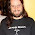## Saturday, September 12, 2009

### Two Puzzles from Down Under

Pat Ballew posted these at Pat'sBlog, and I worked on them last night when I had trouble sleeping. They come from the puzzle corner of the Gazette of the Australian Mathematical Society.

Problem 1. Digital deduction.
The numbers 2^2009 and 5^2009 are written out on a piece of paper in the usual decimal notation. How many digits are on this piece of paper?

Problem 2. Piles of stones.
There are 25 stones sitting in a pile next to a blackboard. You are allowed to take a pile and divide it into two smaller piles of size a and b, but then you must write the number a×b on the blackboard. You continue to do this until you are left with 25 piles, each with one stone. What is the maximum possible sum of the numbers written on the blackboard?

A few of us worked them out in the comments over there. I won't spoil your fun if you're seeing it first here. What I liked about these problems was that from each of them I learned something new that extends well beyond the problem at hand.

#### 6 comments:

1.I just spent like 20 minutes working on problem 1 with logs, forgetting a simple rule of exponents:

(x^a)(y^a) = xy^a
so

(2^2009)(5^2009) = 10^2009, which would be 2010 digits!

(PS I got the same answer using the logs, so at least I know I did that correctly).

Now onto problem 2!

2.Is the answer to Problem 2 = 299?

3.But we're not multiplying them, just counting digits in each one. For example, 2*4 = 8, one digit, 2 and 4 both written down is two digits. Could it be like that? I wasn't sure until I did some work with logs.

Discussion of the answer to the second problem is at Pat'sBlog.

4.Sue: You're right, but there has to be something to the fact that the answer comes out the same!

I wasn't think about using logs for the second problem, but I can see on Pat's blog that's what they're trying to do. Very interesting. Thanks for the challenge!

5.It was fun working on these problems with you over on Pat's blog! And it was nice to see you at SF math circle, too.

I think the logs are just for the first problem; the second problem needs some other method.

6.Yep, no logs in problem number 2.

I loved your insight that the other problem ends up looking like the handshake problem (a revelation to me). Maybe it'll show up this counting course (= SF math circle) you're leading...

Thanks for visiting me here. (I hadn't put two and two together when I saw your comment at Pat's Blog.)